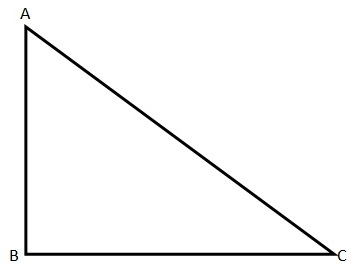# GMAT 800 Problem Solving

 Categories : GMAT 800 Problem Solving, Triangles Find the area of the triangle QRS                           A)    90 cmB)    80 cmC)    110 cmD)    55 cmE)    45 cmSolution• For calculating the area of a triangle, we need to know the basic parameters viz., base and height, and when we substitute them using Area = ½* Base * Height, we will get the area.  • The base can be any side of a given triangle; it can be QR, RS and QS. Depending on the side we choose, the height will be the altitude drawn from the angle opposite to the base.So PQ^2  = QR^2 + PR^2• Let us take... Categories : GMAT 800 Problem SolvingTwo sides of a right angled triangle are 15 cm and 17 cm. Find the length of the third sideI.  8 cm  II. 32 cm    III. Sqrt.514 cm    IV. 514 cmA)    II onlyB)    I onlyC)    I & III onlyD)    II & III onlyE)    I, II & III                    SolutionApart from the math rules, we need to know how to look at these kinds of problems. We can see four options on the top: I, II, III and IV. Then we see five other options A, B, C, D and E.In these types of problems, there will be the chance of more than one option for the given question. Therefore, the possible answers will be one or more among I, II, III and IV. Once we find them, we need to see the options A, B, C, D and E that give the option to choose one or more among I, II, III and IV. So our final answer that we need to mark in the test is among A, B, C, D and E.Math Rules to know for solving this problem: • Pythagoras theorem: The square of... Categories : GMAT 800, GMAT 800 Problem Solving The function g(x) is defined for integers x such that if x is even, g(x) = x/2 and if x is odd, g(x) = x + 5.  Given that g(g(g(g(g(x))))) = 19, how many possible values for x would satisfy this equation?A. 1B. 5C. 7D. 8E. 11Explanation:The easiest way to approach this problem is probably to work backwards, at least until we see a pattern. With g(...) = 19, then we can consider which operation applied to (...).  If it was x/2, then (...)= 38.  38 is even so that is fair.   If it was x + 5, then (...) was 14.  14 is even, so that operation would not have been applied.On paper, you could make a tree, with 19 as the root, and 38 as the first node.  Next consider 38.  38 could have come from 76/2 or 33 + 5.  Two possibilities give us two nodes branching from 38:19  -> 38 -> 76, 33.We can now observe the pattern that with an odd number, it must have come from an even, but an even could come from either of two numbers.Therefore our 76 will branch into 2 numbers, and the 33 into just one.33 -> 6676 -> 73, 152..We can represent this as shown here:...

Get F1GMAT's Newsletters (Best in the Industry)

• Ranking Analysis
• Post-MBA Salary Trends
• Post-MBA Job Function & Industry Analysis
• Post-MBA City Review
• MBA Application Essay Tips
• School Specific Essay Tips
• GMAT Preparation Tips